# CBSE 11TH MATHEMATICS - Online Test

Q1. The next term of the sequence 1, 2, 4, 7,11,…. Is
Explaination / Solution:

here,

where n = 1,2,3,....

so, for 6th term n = 6

Q2. A batsman scores runs in 10 innings as 38,70,48,34,42,55,63,46,54 and 44 , then the mean score is
Explaination / Solution:

Mean (x) =

= 494/10

= 49.4

Q3. The point which divides the joint of ( 1, 2 ) and ( 3,4 ) externally in the ratio 1 : 1 .
Explaination / Solution:

The point which divides the line in the ratio m:n externally is given by x =

Substituting the values we get,

x =  which is undefined.

Q4. Which of the following is a preposition?
Explaination / Solution:

It is a false statement other options are open sentences

Q5. Let P(n)P be a statement and let P(n)⇒P(n+1) for all natural numbers n , then what will the nature of P(n) ?
Explaination / Solution:

Since the statement is conditional statement ( if....then).

Q6. Let  be a statement  , where n is a natural number , then  is true for
Explaination / Solution:

Since for example n = 4 will give LHS as 16 and RHS as 4! = 1.2.3.4= 24

Q7. In how many ways can the letters of the word ‘MATHEMATICS ‘be permuted so that consonants always occur together?
Explaination / Solution:

In the word 'MATHAEMATICS'  there are   7 consonants which are M-2 ,,H-1,C-1,S-1, T-2 .Since they have to occur together we treat them as a single unit.

Now this single unit together with the remaining 4 vowels which are A-2,E-1,and I-1  will account for 5 letters.

Now in these 5 letters we have A is repeating twice  these can be aarranged in   different ways.

Corresponding to each of these arrangements the consonents can be arranged in  different ways.

Hence the number of ways it can be arranged is  =75600

Q8. Length of common chord of the circles and  is
Explaination / Solution: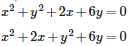Applying the completing the square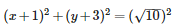Comparing the above equation with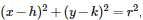we get its center as (-1,-3) and radius (r1)=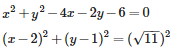Comparing the above equation with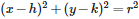we get its center as (2,1) and radius (r2)=

So distance(d) between the centers isunits
After applying general formula of length of common chord as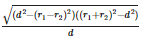and putting the values of r1, r2 and d we get length as
.

Q9. In a triangle ABC, if A =  and B =  then b + 2 c is equal to
Explaination / Solution: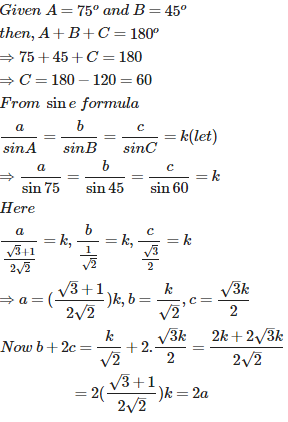Q10. The direction cosines of X -axis are
Explaination / Solution:

As we know that if a lines makes angles b and c with X-axis , Y-axis and Z-axis respectively then direction cosines are given by  cos a , cos b ,cos c >

In our case line is X-axis itself which we know makes angle of 0${}^{\circ }$ , 90${}^{\circ }$ , 90${}^{\circ }$ with  X-axis , Y-axis and Z-axis respectively then direction cosine will be

<cos 0${}^{\circ }$ , cos 90${}^{\circ }$, cos 90${}^{\circ }$>

= < 1 0 0 >